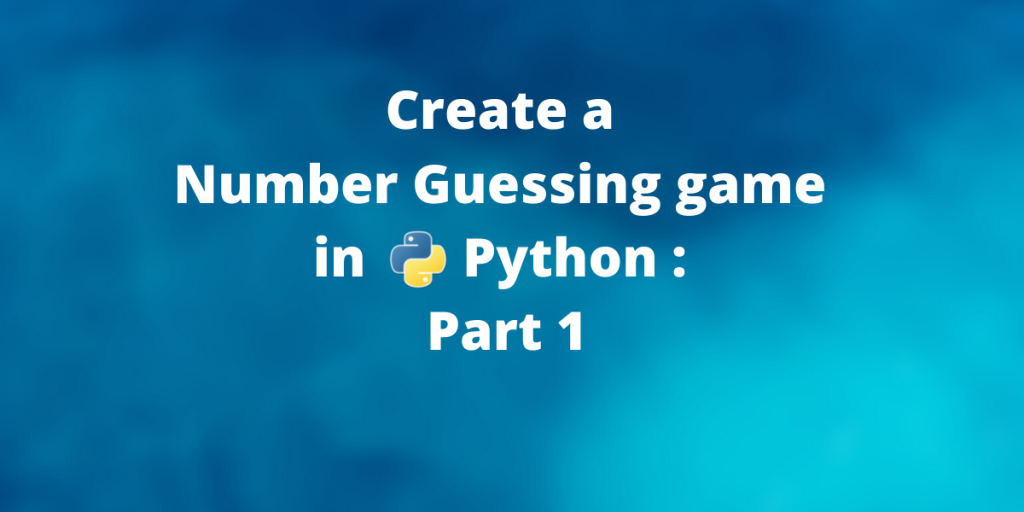# Create a Number Guessing game in Python: Part 1 (Command Line)In this tutorial, we will be creating a random number guessing game using standard python libraries. This tutorial is divided into two parts- The Command Line Interface(CLI) and the Graphical User Interface(GUI).

This the first part of the tutorial where we implement the game and run it in a command line without any graphical eye candy. The next part of the tutorial is an extension of this tutorial for adding a graphical interface to the game. This is a beginner level project but a very basic understanding of python is expected.

## Understanding the rules of the number guessing game

Before we proceed with coding our application it is very important to clearly understand what the application should do and how it is expected to behave.

So we first lay out the basic rules of the game:

1. The computer will guess a number between 1 and 1000 and and you need to guess the number.
2. Unlimited number of retries will be provided till you guess the correct number. The one with least number of retries wins the game.
3. The computer will give a hint to the user in case the player chooses a wrong number – The computer will tell the player if target is within 0 and the number guessed by the user. For eg: If you choose 78 and the number is 45, the target number(45) lies between 0 and 78, so the computer will prompt accordingly.

## Creating a Python number guessing game

So let us the dive into the implementation of the code.

Note: Though this code-base is implemented just in CLI, it will serve as a backbone for the GUI version of the game.

### Generating a random number between 1 and 1000

To generate a random number between 1 and 1000 we will be using the randint() function from the random library in python.

```# Import the random module
import random

# Computer chooses a random number between 1 and 1000
target = random.randint(1, 1000)
```

### Inputing a number from the user

To input a number a from the user we use will be using the input() function

```# Input the guess from the user
choice = int(input("Enter your guess: "))
```

Now that we have the user input we can compare the two number and display a single output. For the game to continue until the user chooses the correct choice, we have to put everything in a loop.

### Looping the entire process

There are three more things we need to take care of:

1. Generate the correct prompts for guesses and hints: This can be implemented using nested if-else blocks.
2. Count the number of retires: Take a counter variable and increase it every time the player makes a wrong choice.
3. Repeat all operations until the correct guess is made: Enclose all the operations in an infinite loop that only breaks when the correct guess is made.

We solve the three sub problems together is this block of code:

```# Counter Variable
retries = 0

while(True):

# Taking user choice
choice = int(input("Enter your choice: "))
retries += 1

# Wrong guess
if target != choice:

print("Wrong Guess!! Try Again")

# Hint
if target < choice:
print("The required number lies between 0 and {}".format(choice))
else:
print("The required number lies between {} and 1000".format(choice))

# Correct choice
else:
print("You guessed the  correct number after {} retries".format(retries))
# User guessed the correct value
# So let's end the infinite loop
break;
```

### Final Python code for our number guessing game

Putting all the blocks of code together, the final Python program looks like:

```# Import the random module
import random

# Computer chooses a random number between 1 and 1000
target = random.randint(1, 1000)

retries = 0

while(True):

# Taking user choice
choice = int(input("Enter your choice: "))
retries += 1

# Wrong guess
if target != choice:

print("Wrong Guess!! Try Again")

# Hint
if target < choice:
print("The required number lies between 0 and {}".format(choice))
else:
print("The required number lies between {} and 1000".format(choice))

# Correct choice
else:
print("You guessed the correct number after {} retries".format(retries))
# User guessed the correct value
# So let's end the infinite loop
break;

```

To run this program save it in a python file. We have saved it by the name “guessing_game.py”. You choose any other name but make sure that the extension is .py which indicates that the file is python file.

Run the python file from the command line. You can execute it from your favourite IDE if you like.

```python3 guessing_game.py
```